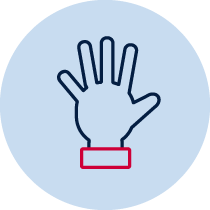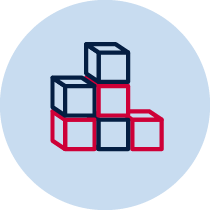# 2D – Drawing diagrams from descriptionsPractical Build/make Resource required Verbal recording Take photo Collaborative

## Measurement and Geometry – 2D space

• describes mathematical situations and methods using everyday and some mathematical language, actions, materials, diagrams and symbols MA1-1WM
• uses objects, diagrams and technology to explore mathematical problems MA1-2WM
• supports conclusions by explaining or demonstrating how answers were obtained MA1-3WM
• manipulates, sorts, represents, describes and explores two-dimensional shapes, including quadrilaterals, pentagons, hexagons and octagons MA1-15MG
• represents and describes the positions of objects in everyday situations and on maps MA1-16MG

## Content

Two-dimensional space 1

Recognise and classify familiar two-dimensional shapes using obvious features (ACMMG022)

• Describe features of two-dimensional shapes using the terms ‘side’ and ‘vertex’ (communicating)
• Select a shape from a description of its features (reasoning)

Two-dimensional space 2

Describe and draw two-dimensional shapes, with and without the use of digital technologies (ACMMG042)

Identify and describe half-turns and quarter-turns (ACMMG046)

Position 1

Give and follow instructions to position objects in models and drawings, eg 'Draw the bird between the two trees'

• give and follow simple directions using a diagram or description (Communicating)
• use a diagram to give simple directions (Communicating)

Position 2

Describe the positions of objects in models, photographs and drawings

Make simple model from memory, photographs, drawings or descriptions, eg students make a model of their classroom

## National Numeracy Learning Progression Mapping to the NSW mathematics syllabus

When working towards the outcome MA1-15MG the sub-elements (and levels) of Interpreting fractions (InF1) and Understanding geometric properties (UGP2-UPG4) describe observable behaviours that can aid teachers in making evidence-based decisions about student development and future learning.

When working towards the outcome MA1-16MG the sub-elements (and levels) of Interpreting fractions (InF1) and Positioning and locating (PoL2-PoL3) describe observable behaviours that can aid teachers in making evidence-based decisions about student development and future learning.

## Materials

• Paper and pencils
• Geometric pattern blocks
• Optional – video recording device

## Teacher instructions

The purpose of this task is to gauge students' understanding of 2D shape concepts such as:

• recognise familiar two-dimensional shapes using obvious features
• classify familiar two-dimensional shapes using obvious features
• describe features of two-dimensional shapes using the terms ‘side’ and ‘vertex’
• describe and draw two-dimensional shapes

Part A

As a barrier task, students sit back to back and describe a 2D shape to their partner who attempts to draw and guess the shape. For example, my shape has 4 sides, 4 vertices and 2 sets of parallel lines. 1 set is vertical and the other set is horizontal. All sides are equal.

Prompting questions

• What did your partner say that helped you draw your shape?
• Were there any other shapes that could have come from the description?

Part B

Students draw diagrams from the teacher’s descriptions.

Draw the diagrams that might be represented by these descriptions. There is more than one possibility for each description.

'A triangle is drawn beside the circle, on the right-hand side, just touching the circle. A square is drawn above the triangle. A line is drawn from 1 vertex of the square to 1 vertex of the triangle.'

'A triangle is drawn next to the pentagon, on the left-hand side and just touching the pentagon. A hexagon is drawn below the triangle. A line is drawn from 1 vertex of the hexagon to 1 vertex of the triangle.'

Enabling prompt

If students are unable to identify and/or draw the two-dimensional shapes, provide pattern blocks for students to trace.

Extending prompt

Students create their own instructions for a diagram. One student shares their description for their diagram, while another student attempts to draw it. The descriptions could also be written and/or spoken and recorded on a device. Peer feedback is then provided, so that the instructions can be refined and improved.

Students should be able to communicate using the following language: shape, circle, triangle, quadrilateral, square, rectangle, pentagon, hexagon, octagon, features, side, vertex (vertices), vertical, horizontal, parallel, two-dimensional shape (2D shape), turn, full-turn, half-turn, quarter-turn, clockwise, anti-clockwise, left, right.

Students should also be able to communicate using the following language: position, left, right, directions, turn.

In Early Stage 1, students used the terms 'left' and 'right' to describe position in relation to themselves. In Stage 1, students use the terms 'left' and 'right' to describe position from the perspective of a person facing in the opposite direction.

## Student instructions

Part A

Sit back to back with a partner. Choose a shape and use mathematical language to describe it’s features to your partner. Your partner will attempt to:

Part B

Draw the diagrams to represent these descriptions. There is more than one possibility for each description.

“A triangle is drawn on the right-hand side of a circle, just touching the circle. A square is drawn above the triangle. A line is drawn from 1 vertex of the square to 1 vertex of the triangle.”

“A triangle is drawn on the left-hand side of a pentagon, just touching the pentagon. A hexagon is drawn below the triangle. A line is drawn from 1 vertex of the hexagon to 1 vertex of the triangle.”

Share your diagrams with a partner and see if they are the same. Discuss the reasons why your diagrams are the same or different.

There could be an opportunity for the students to give the teacher feedback on the instructions which would allow further questioning to gauge student understanding.

Extending prompt

## Possible areas for further exploration

• mathematical language
• full-turn, half-turn, quarter-turn clockwise/anti-clockwise
• recognising and classifying two-dimensional shapes

## Where to next?

• Repeat the activity with strategic grouping (differentiation)
• Utilising digital drawing tools to complete similar tasks
• Describing a shape in a different orientation (upside down)
• Describe a pattern of shapes